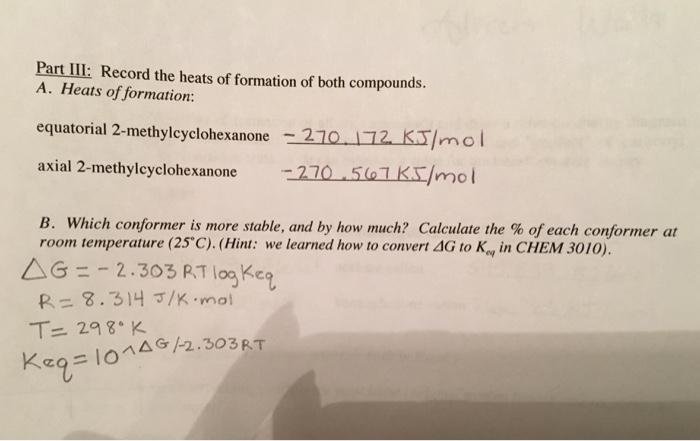# Question & Answer: Part III: Record the heats of formation of both compounds. A. Heats of formation: equatorial…..

If someone could show me how to work out part Bbstep by step that would be awesome. Thank you!Part III: Record the heats of formation of both compounds. A. Heats of formation: equatorial 2-methylcyclohexanone axial 2-methylcyclohexanone -210-17aKJ/mol -270-501Ks/mol B. which conformer is more stable, and by how much? Calculate the % of each conformier at rorn temperature (25°C). (Hint: we learned how to conver△G to Kol in CHEM 3010). △G:-2.303 RT logKeg R= 8.314 J/K-mol eg

Given that;

Equatorial 2-methyl cyclohexanone < = > axial 2-methyl cyclohexanone

Don't use plagiarized sources. Get Your Custom Essay on
Question & Answer: Part III: Record the heats of formation of both compounds. A. Heats of formation: equatorial…..
GET AN ESSAY WRITTEN FOR YOU FROM AS LOW AS \$13/PAGE

K = [ axial 2-methyl cyclohexanone ]/ Equatorial 2-methyl cyclohexanone

Now calculate the ΔH fro this conversation as follows

ΔH= ΔH product – ΔH reactant

= -270.567 KJ/ mole – (- 270.172 KJ/ mole)

= -0.395 KJ/ moles

= – 395 J / mole

In this case

Heats of formation

ΔH ≈ ΔG

ΔG =-RTlnK

Here R = 8.3145 J / mole K

T= 25C= 298K

K = e-ΔG/RT

K = e -(-395 J / moles )/ (8.3145 )(298)

K = e ^0.159

= 1.44

K = [ axial 2-methyl cyclohexanone ]/ Equatorial 2-methyl cyclohexanone

Thus axial 2-methyl cyclohexanone / Equatorial 2-methyl cyclohexanone = 1.44/1

axial 2-methyl cyclohexanone   = 1.44 * Equatorial 2-methyl cyclohexanone

assume that Equatorial 2-methyl cyclohexanone is 1

% of axial 2-methyl cyclohexanone = 1.44/2.44 ]*100

= 59.0%

And

% of Equatorial 2-methyl cyclohexanone = 1.00/2.44 ]*100

= 41.0%